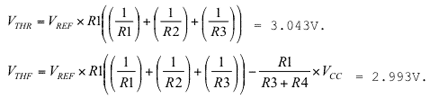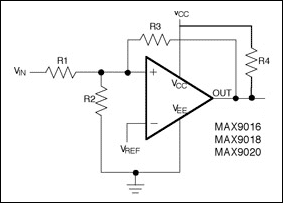# Adding Extra Hysteresis to Comparators

### Abstract

Analog comparators are always in the shadow of their more popular cousin, the ubiquitous op amp. Designers can draw on a wealth of application notes for op amps, but not so for comparators. One consequence of that scarcity is that customers call the Maxim applications line for help when adding comparator hysteresis. This note explains how to add hysteresis to some common comparator circuits, providing increased noise immunity and stability.

A discussion of comparator hysteresis begins with a definition of the word. Like so many other scientific words, it is derived from the Greek. In this case, it means to lag behind or follow, or to offer resistance to change from a previous state. We use it in engineering to describe an operation that is not symmetrical, i.e., its path from A to B is not the same as from B to A. Hysteresis is found in the phenomena of magnetism and non-plastic deformation, and in electronic circuits such as comparators.

Hysteresis is designed into most comparators, usually with a value of 5mV to 10mV. Internal hysteresis helps the comparator avoid oscillation due to small amounts of parasitic feedback. Though sufficient to prevent the comparator from self-oscillating, such internal hysteresis can easily be swamped by any external noise of greater amplitude. In that case, you can improve performance by adding hysteresis externally.

First, consider the transfer function for an ideal comparator without internal hysteresis (Figure 1). In contrast, the transfer characteristic for a real comparator (Figure 2) shows an output change that requires an increase of about 2mV in the input voltage (VIN).For open-loop op amps, which are often used as comparators, a small amount of noise or interference combined with the input signal can cause undesirable rapid changes between the two output states (Figure 3). Substituting a comparator with hysteresis can prevent the rapid output changes and oscillation. Or, you can create external hysteresis by applying positive feedback to a comparator. Because positive feedback guarantees a fast output transition from one state to the other, the comparator output spends a negligible amount of time in the indeterminate state.As an example, consider the simple circuit in Figure 4, with a transfer characteristic as shown in Figure 5, with a voltage ramp starting from zero applied to the comparator's inverting input. Resistor divider R1-R2 provides positive feedback. When the input begins to increase from point 1 (Figure 6), the output is at VCC level and remains there until the input passes the positive threshold: VTH+ = VCCR2/(R1 + R2). At that point, the output changes drastically from VCC to VSS, because the inverting input is more positive than the noninverting input. The output remains low until the input passes the new threshold at point 5: VTH- = VSSR2/(R1 + R2). At that time, the output switches immediately to VCC, because the positive (noninverting) input has a higher potential than the inverting one.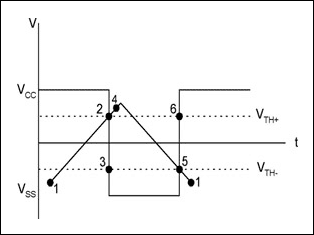The transfer function VOUT vs. VIN for the circuit in Figure 4 shows that the output changes in response to an input change of at least 2VTH. Thus, unlike the response of Figure 3 (an op amp with no hysteresis), any small amount of noise or interference less than 2VTH cannot cause a rapid output change. For a particular application, the positive and negative threshold voltages can be set to desired values by a suitable choice of feedback network.

Other configurations are available for adding hysteresis with different threshold voltages. The circuit in Figure 7 uses two MOSFETs and a resistor network to adjust or shift the threshold level in either direction. Unlike the comparator output in Figure 4, this one is not loaded with a feedback resistor network. The output responds to input changes as shown in Figure 8.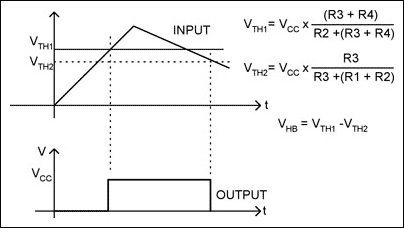Different output configurations internal to the comparator require different implementations of the external hysteresis. Comparators with internal push-pull outputs, for example, can employ a positive-feedback resistor directly between the output and noninverting input. A resistor divider network applies the input signal to the comparator's noninverting input, and the inverting input is fixed at some reference level (Figure 9).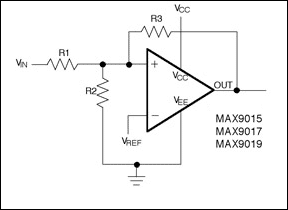As noted, a comparator with internal hysteresis presents one trip point for the rising input voltage (VTHR) and one for the falling input voltage (VTHF), corresponding to VTH1 and VTH2 in Figure 8. The difference between these trip points is the hysteresis band (VHB). When the comparator's input voltages are equal, the hysteresis causes one input to move quickly past the other, thereby removing the input voltages from the region where oscillation occurs. Figure 10 illustrates the behavior of a comparator with fixed voltage applied to the inverting input and a varying voltage applied to the noninverting input. (Swapping the inputs produces a figure that is similar, but with inverted output.)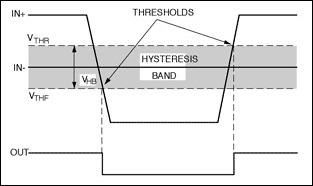Resistor values for the feedback-divider network are based on simple calculations of the two known cases in which the output is at one extreme or the other (the two supply rails).

Consider a comparator with internal hysteresis of 4mV and a push-pull output configuration—such as the Maxim MAX9015, MAX9017, and MAX9019. These comparators are intended for single-supply systems in which the two rails are VCC and 0V. The following procedure lets you select or calculate the components based on the requirements and on given data such as supply rail voltages, hysteresis band voltage (VHB), and reference voltage (VREF):

### Step 1.

Select R3. The current through R3 at the trip point is (VREF - VOUT)/R3. Considering the two possible output states in solving for R3 yields two formulas:
R3 = VREF/IR3 and R3 = (VCC - VREF)/IR3.
Choose the smaller of the two resulting resistor values. For example, for VCC = 5V, IR3 = 0.2µA, and a MAX9117 comparator (VREF = 1.24V), the two resistor values are 6.2MΩ and 19MΩ. For R3 you should therefore choose the standard value 6.2MΩ.

### Step 2.

Choose the hysteresis band required (VHB). For this example, choose 50mV.### Step 3.

Calculate R1 according to the following equation:
For this example, insert the value### Step 4.

Choose the trip point for VIN rising (VTHR), such that:This is the threshold voltage at which the comparator switches its output from low to high as VIN rises above the trip point. For this example, choose VTHR = 3V.

### Step 5.

Calculate R2 as follows:For this example, choose a standard value of 44.2kΩ.

### Step 6.

Verify the trip voltages and hysteresis as follows: VIN rising = 2.992V, which is equivalent to VREF times R1 divided by the parallel combination of R1, R2, and R3:VIN falling = 2.942V. Therefore, hysteresis = VTHR - VTHF = 50mV.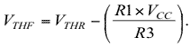Finally, comparators with open-drain outputs and a built-in hysteresis band of 4mV (MAX9016, MAX9018, MAX9020) require an external pull-up resistor (Figure 11). Additional hysteresis can be generated using positive feedback, but the formulas differ slightly from those for push-pull outputs. Hysteresis = VTHR - VTHF = 50mV.

Use the following procedure to calculate resistor values:

### Step 1.

Select R3. Input bias current at IN_+ is less than 2nA, so to minimize errors caused by the input bias current, the current through R3 should be at least 0.2µA. Current through R3 at the trip point is (VREF - VOUT)/R3. Considering the two possible output states in solving for R3 yields two formulas:
R3 = VREF/IR3 and R3 = [(VCC - VREF)/IR3] - R4.
Use the smaller of the two resulting resistor values. For example, for VCC = 5V, IR3 = 0.2µA, R4 = 1MΩ, and a MAX9118 comparator (VREF = 1.24V), the two resistor values are 6.2MΩ and 18MΩ. Therefore, for R3 choose the standard value of 6.2MΩ.

### Step 2.

Choose the hysteresis band required (VHB).

### Step 3.

Calculate R1 according to the following equation:For this example, insert the value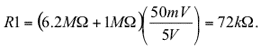### Step 4.

Choose the trip point for VIN rising (VTHR) such that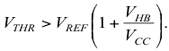VTHR is the threshold voltage at which the comparator switches its output from low to high, as VIN rises above the trip point. For this example, choose VTHR = 3V.

### Step 5.

Calculate R2 as follows:For this example, choose a standard value of 49.9kΩ.

### Step 6.

Verify the trip voltages and hysteresis as follows: